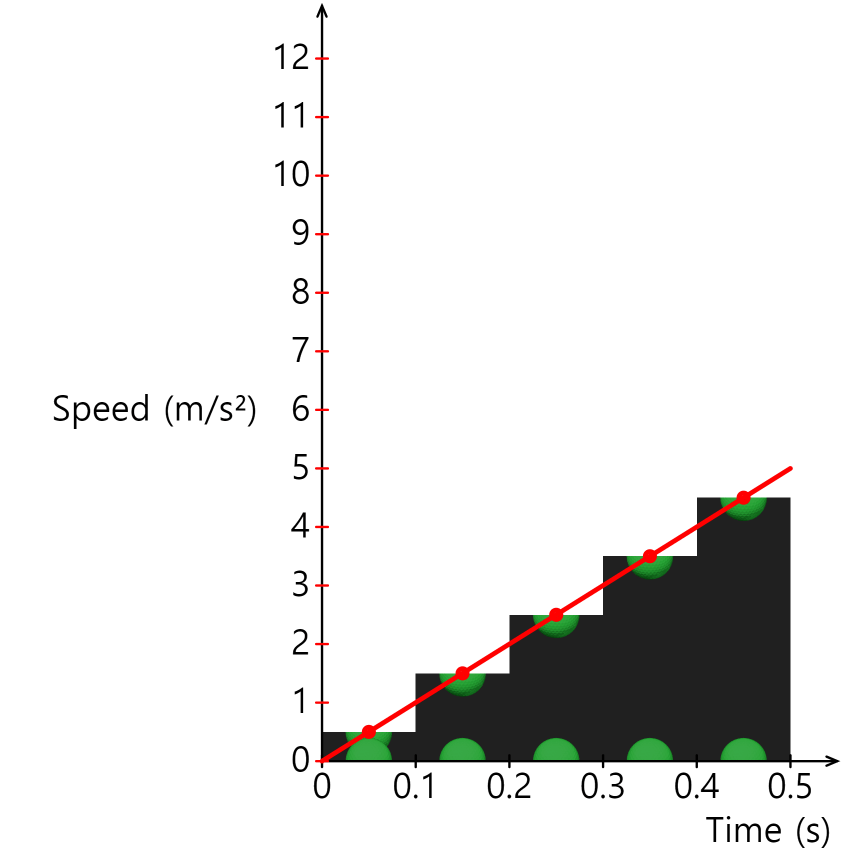# Analysis of Free Fall Motion

* Please refer to the text below for how to make a graph.

## Free fall motion

If you hold the ball in your hand and release it, it will fall.
In the absence of air resistance, the only force acting on the ball while it is falling is 'gravity.'

If you take pictures of free-falling balls at equal time intervals, the distance between the balls gradually increases. The graph of distance traveled over time can be expressed as follows.The distance between the balls is the distance traveled by the balls in a certain amount of time, meaning the speed. Therefore, it can be seen that a ball moving in free fall has a constant increase in speed with time. The time-speed graph can be expressed as:Analysis of the time-speed graph of free-fall motion reveals that the speed increases by '9.8 m/s' every second, where '9.8' is called the 'gravity acceleration constant' of the Earth.
Some textbooks calculate 9.8 by rounding it to 10. (Convenience of calculation process)